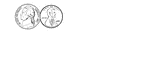### 6 Cents

Groups of change with totals from 1 to 100 cents using the least amount of coins.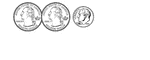### 60 Cents

Groups of change with totals from 1 to 100 cents using the least amount of coins.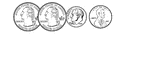### 61 Cents

Groups of change with totals from 1 to 100 cents using the least amount of coins.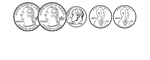### 62 Cents

Groups of change with totals from 1 to 100 cents using the least amount of coins.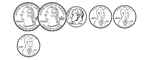### 63 Cents

Groups of change with totals from 1 to 100 cents using the least amount of coins.### 64 Cents

Groups of change with totals from 1 to 100 cents using the least amount of coins.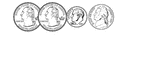### 65 Cents

Groups of change with totals from 1 to 100 cents using the least amount of coins.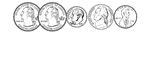### 66 Cents

Groups of change with totals from 1 to 100 cents using the least amount of coins.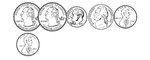### 67 Cents

Groups of change with totals from 1 to 100 cents using the least amount of coins.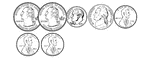### 68 Cents

Groups of change with totals from 1 to 100 cents using the least amount of coins.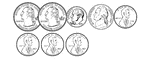### 69 Cents

Groups of change with totals from 1 to 100 cents using the least amount of coins.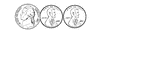### 7 Cents

Groups of change with totals from 1 to 100 cents using the least amount of coins.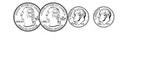### 70 Cents

Groups of change with totals from 1 to 100 cents using the least amount of coins.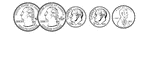### 71 Cents

Groups of change with totals from 1 to 100 cents using the least amount of coins.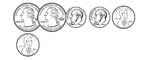### 72 Cents

Groups of change with totals from 1 to 100 cents using the least amount of coins.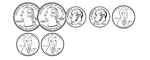### 73 Cents

Groups of change with totals from 1 to 100 cents using the least amount of coins.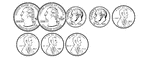### 74 Cents

Groups of change with totals from 1 to 100 cents using the least amount of coins.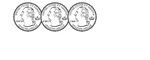### 75 Cents

Groups of change with totals from 1 to 100 cents using the least amount of coins.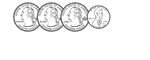### 76 Cents

Groups of change with totals from 1 to 100 cents using the least amount of coins.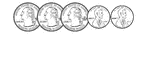### 77 Cents

Groups of change with totals from 1 to 100 cents using the least amount of coins.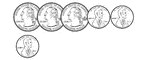### 78 Cents

Groups of change with totals from 1 to 100 cents using the least amount of coins.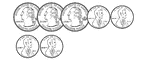### 79 Cents

Groups of change with totals from 1 to 100 cents using the least amount of coins.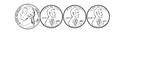### 8 Cents

Groups of change with totals from 1 to 100 cents using the least amount of coins.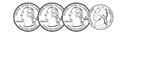### 80 Cents

Groups of change with totals from 1 to 100 cents using the least amount of coins.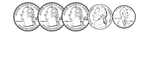### 81 Cents

Groups of change with totals from 1 to 100 cents using the least amount of coins.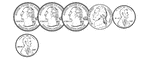### 82 Cents

Groups of change with totals from 1 to 100 cents using the least amount of coins.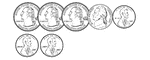### 83 Cents

Groups of change with totals from 1 to 100 cents using the least amount of coins.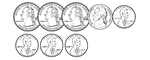### 84 Cents

Groups of change with totals from 1 to 100 cents using the least amount of coins.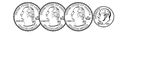### 85 Cents

Groups of change with totals from 1 to 100 cents using the least amount of coins.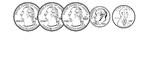### 86 Cents

Groups of change with totals from 1 to 100 cents using the least amount of coins.### 87 Cents

Groups of change with totals from 1 to 100 cents using the least amount of coins.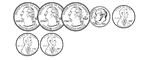### 88 Cents

Groups of change with totals from 1 to 100 cents using the least amount of coins.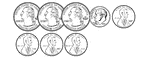### 89 Cents

Groups of change with totals from 1 to 100 cents using the least amount of coins.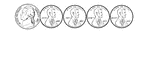### 9 Cents

Groups of change with totals from 1 to 100 cents using the least amount of coins.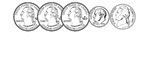### 90 Cents

Groups of change with totals from 1 to 100 cents using the least amount of coins.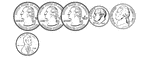### 91 Cents

Groups of change with totals from 1 to 100 cents using the least amount of coins.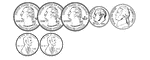### 92 Cents

Groups of change with totals from 1 to 100 cents using the least amount of coins.### 93 Cents

Groups of change with totals from 1 to 100 cents using the least amount of coins.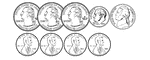### 94 Cents

Groups of change with totals from 1 to 100 cents using the least amount of coins.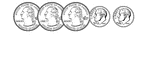### 95 Cents

Groups of change with totals from 1 to 100 cents using the least amount of coins.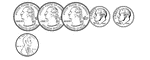### 96 Cents

Groups of change with totals from 1 to 100 cents using the least amount of coins.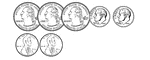### 97 Cents

Groups of change with totals from 1 to 100 cents using the least amount of coins.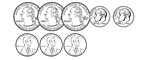### 98 Cents

Groups of change with totals from 1 to 100 cents using the least amount of coins.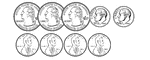### 99 Cents

Groups of change with totals from 1 to 100 cents using the least amount of coins.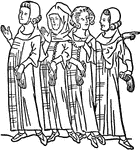### Clerks

An illustration of a group of clerks.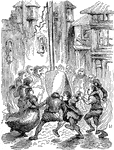### Crowd Fighting

An illustration of a crowd fighting.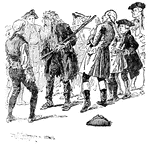### Crowd of Men

A group of adult males surrounding a ragged woodsman who is holding a gun.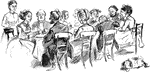### Dinner Party

An illustration of a group of men and women attending a dinner party.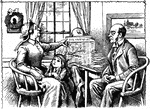### Family Reading Paper

An illustration of a family reading a newspaper together around the table.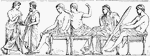### Frieze

"Group from the Eastern frieze of the Parthenon." —D'Anvers, 1895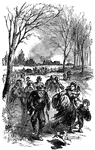### Fugitives Before the Approach of a Hostile Army

Group of people leaving a rural area.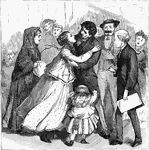### Greeting Friends

An illustration of a group of friend greeting each other.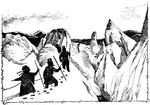### Group Hiking

An illustration of a group hiking through the mountains.### Group in Graveyard

An illustration of a group of people standing in a graveyard.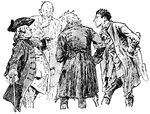### Group of men

A group of adult males huddled together talking.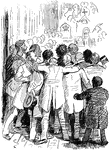### Group of Men

An illustration of a group of men from behind.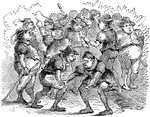### Group of Men

An illustration of a group of men.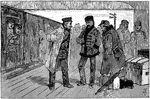### Group of Men Waiting at Train Station

An illustration of a group of men waiting at a train station.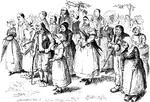### Group of Townspeople

An illustration of a group of townspeople from Sainte-Anne-d'Auray as pictured at their pardon in 1623.### Group of Women

An illustration of a group of women standing together.